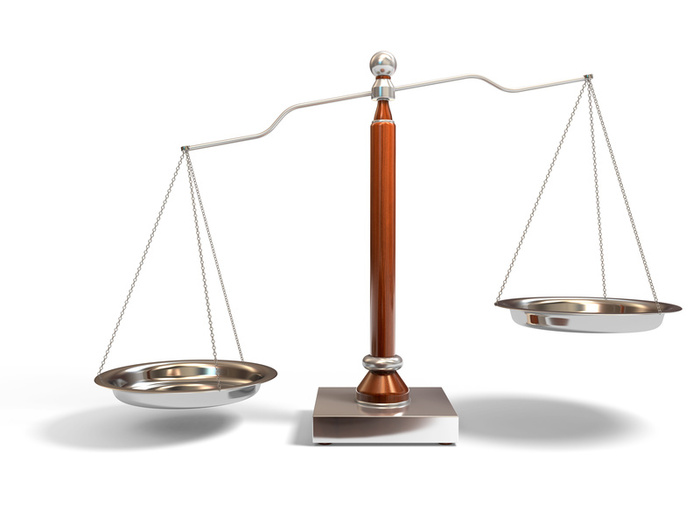# Maths interview question: 8 balls with 1 weighing more than the othersThis is another common interview question, but appropriate for primary school.

You have 8 balls which look identical. 7 of them have the same weight, while 1 is heavier. How can you find the heavier ball by using a balance with only 2 weighings?

The most common way (but this will require 3 weighings) would be to divide the 8 balls into 2 groups of 4. The heavier group will contain the heavier ball. Divide this group of 4 into another 2 groups of 2. And so on.

The solution is to balance only 6 out of the 8 balls.

• Divide the 6 balls into two groups.
• If the heavy ball isn’t in one of those 6 balls, then you’re left with 2 balls. The heavier ball can be determined with one more weighing.
• If one side is heavier, the heavier ball must be one of the three balls. Pick any two balls to balance. If they weigh the same, then the ball left out will be the heavier one.  If not, you know which one is heavier.